Next: Wave-Front Propagation Up: Wave Propagation in Uniform Previous: Wave Propagation in Magnetized

# Wave Propagation in Dispersive Media

Let us investigate the propagation of electromagnetic radiation through a general dispersive medium by studying a simple one-dimensional problem. Suppose that our dispersive medium extends from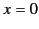, where it interfaces with a vacuum, to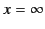. Suppose, further, that an electromagnetic wave is incident normally on the interface such that the field quantities at the interface only depend on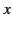and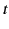. The wave is then specified as a given function ofat. Because we are not interested in the reflected wave, let this function,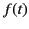, say, specify the wave amplitude just inside the surface of the dispersive medium. Suppose that the wave arrives at this surface at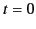, and that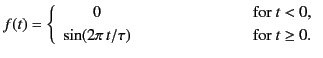(853)

How does the wave subsequently develop in the region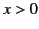? In order to answer this question, we must first of all decomposeinto harmonic components of the form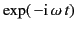(i.e., Fourier harmonics). Unfortunately, if we attempt this using only real frequencies,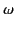, then we encounter convergence difficulties, becausedoes not vanish at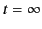. For the moment, we can circumvent these difficulties by only considering finite (in time) wave-forms. In other words, we now imagine that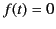for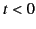and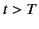. Such a wave-form can be thought of as the superposition of two infinite (in time) wave-forms, the first beginning at, and the second at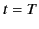with the opposite phase, so that the two cancel for all time.

According to standard Fourier transform theory,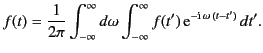(854)

Becauseis a real function ofthat is zero forand, we can write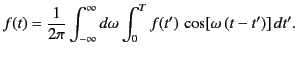(855)

Finally, it follows from symmetry (in) that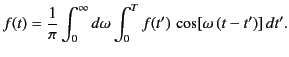(856)

Equation (854) yields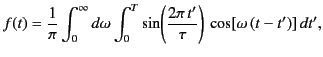(857)

or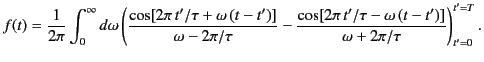(858)

Let us assume, for the sake of simplicity, that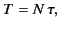(859)

where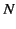is a positive integer. This ensures thatis continuous at. Equation (859) reduces to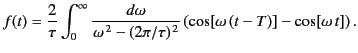(860)

This expression can be written(861)

or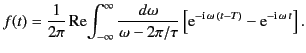(862)

It is not entirely obvious that Equation (863) is equivalent to Equation (862). However, we can easily prove that this is the case by taking Equation (863), and then using the standard definition of a real part (i.e., half the sum of the quantity in question and its complex conjugate) to give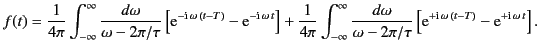(863)

Replacing the dummy integration variableby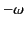in the second integral, and then making use of symmetry, it is easily seen that the previous expression reduces to Equation (862).

Equation (862) can be written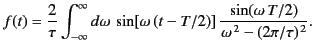(864)

Note that the integrand is finite at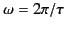, because, at this point, the vanishing of the denominator is compensated for by the simultaneous vanishing of the numerator. It follows that the integrand in Equation (863) is also not infinite at, as long as we do not separate the two exponentials. Thus, we can replace the integration along the real axis through this point by a small semi-circle in the upper half of the complex plane. Once this has been done, we can deform the path still further, and can integrate the two exponentials in Equation (863) separately: that is,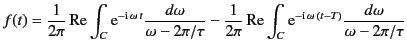(865)

The contour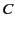is sketched in Figure 6. Note that it runs from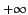to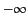, which accounts for the change of sign between Equations (863) and (866).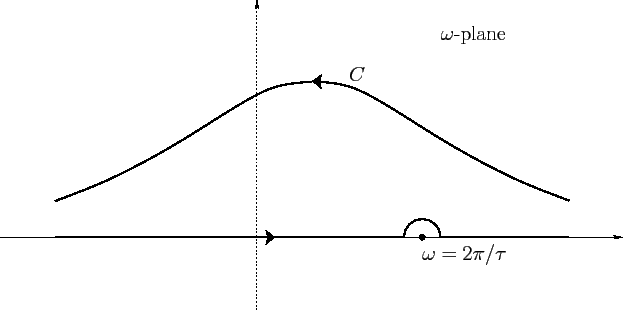We have already mentioned that a finite wave-form that is zero forandcan be through of as the superposition of two out of phase infinite wave-forms, one starting at, and the other at. It is plausible, therefore, that the first term in the previous expression corresponds to the infinite wave form starting at, and the second to the infinite wave form starting at. If this is the case then the wave-form (854), which starts atand ends at, can be written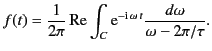(866)

Let us test this proposition. In order to do this, we must replace the original path of integration,, by two equivalent paths.

First, consider. In this case,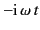has a negative real part in the upper half-plane that increases indefinitely with increasing distance from the axis. Thus, we can replace the original path of integration by the path. (See Figure 7.) If we letapproach infinity in the upper half-plane then the integral clearly vanishes along this path. Consequently,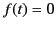(867)

for.

Next, consider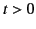. Nowhas a negative real part in the lower half-plane, so the exponential vanishes at infinity in this half-plane. If we attempt to deformto infinity in the lower half-plane then the path of integration catches'' on the singularity of the integrand at. (See Figure 7.) The path of integration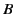therefore consists of three parts: 1) the part at infinity,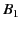, where the integral vanishes due to the exponential factor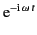; 2)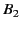, the two parts leading to infinity, which cancel one another, and, thus, contribute nothing to the integral; 3) the path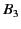around the singularity. This latter contribution can easily be evaluated using the Cauchy residue theorem: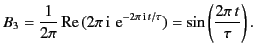(868)

Thus, we have proved that expression (867) actually describes a wave-form, beginning at, whose subsequent motion is specified by Equation (854).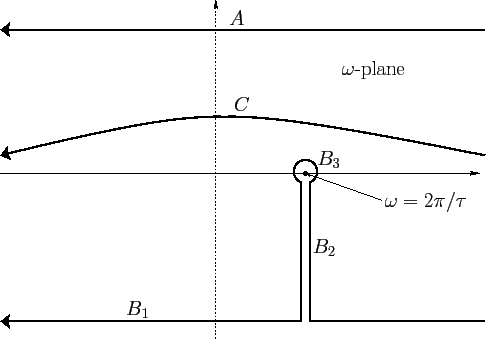Equation (867) can immediately be generalized to give the wave motion in the region: that is,(869)

This follows from standard wave theory, because we know that an unterminated wave motion atof the formtakes the form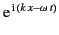after moving a distanceinto the dispersive medium, provided that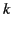andare related by the appropriate dispersion relation. For a dielectric medium consisting of a single resonant species, this dispersion relation is written [see Equation (785)]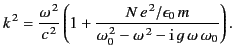(870)Next: Wave-Front Propagation Up: Wave Propagation in Uniform Previous: Wave Propagation in Magnetized
Richard Fitzpatrick 2014-06-27